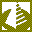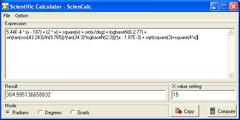Search
Software
Options for Scientific Calculator - ScienCalc
Scientific Calculator - ScienCalcScienCalc is a convenient and powerful scientific calculator. ScienCalc calculates mathematical expression. It supports the common arithmetic operations (+, -, *, /) and parentheses.

The program contains high-performance arithmetic, trigonometric, hyperbolic and transcendental calculation routines. All the function routines therein map directly to Intel 80387 FPU floating-point machine instructions.

Find values for your equations in seconds:
Scientific calculator gives students, teachers, scientists and engineers the power to find values for even the most complex equation set. You can build equation set, which can include a wide array of linear and nonlinear models for any application:

*Linear equations
*Polynomial and rational equations
*Nonlinear exponential, logarithmic and power equations
*Pre-defined mathematical and physical constants

Understandable and convenient interface:
A flexible work area lets you type in your equations directly. It is as simple as a regular text editor. Annotate, edit and repeat your calculations in the work area. You can also paste your equations into the editor panel.
Example of mathematical expression:
5.44E-4 * (x - 187) + (2 * x) + square(x) + sin(x/deg) + logbaseN(6;2.77)
History of all calculations done during a session can be viewed. Print your work for later use. Comprehensive online help is easily accessed within the program.

Features:
--------
*Scientific calculations
*Unlimited expression length
*Parenthesis compatible
*Scientific notation
*More than 35 functions
*More than 40 constants
*User-friendly error messages
*Simple mode (medium size on desktop)
*Paste expressions into ScienCalc
*Comprehensive documentation
*All the benefits that Windows bestows, such as multi-tasking and print formatting are available